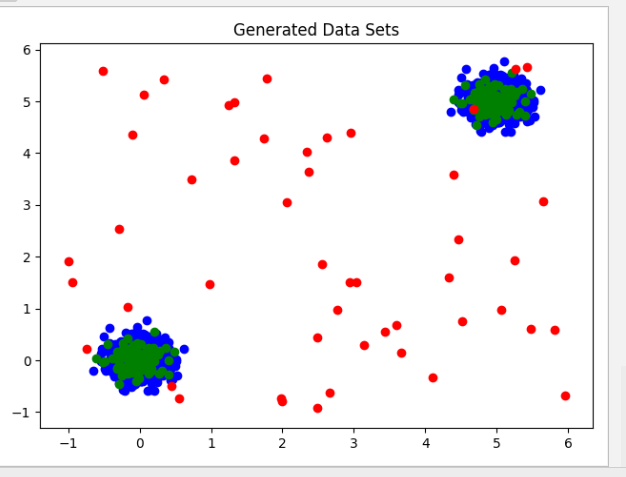# Anomaly Detection: Isolation Forest Algorithm

AWS Certified MLS | 21 Nov 2019
Tags: anomaly-detection, isolation-forest

## Defining the Anomaly Detection Problem

• Data is set of vectors in d-dimensional space
• $x_1$, … $x_n$ each $x_i$ $\epsilon$ $R^d$
• Mixture of nominal points and anomaly points
• Anomaly points are generated by different generative process than the normal points

Solutions

• Supervised
• Training data labeled with “nominal” or “anomaly”
• Clean
• Training data are all “nominal”, test data contaminated with “anomaly” points
• Unsupervised
• Training data consists of mixture of “nominal” and “anomaly” points

### Methods to Resolve Anomaly Detection Problem

• Density Based
• DBSCAN
• LOF
• Distance Based
• KNN
• K-MEANS
• Regression hyperplane distance
• Parametric
• GMM
• Single Class SVMs
• Extreme value theory

### Well Defined Anomaly Distribution Assumption

• WDAD: the anomalies are drawn from a well-defined probability distribution

• The WDAD assumption is often risky

• adversarial situations (fraud, insider threats, cyber security)
• diverse set of potential causes
• user’s notion of anomaly changes with time

## Isolation Forest Algorithm

• Builds an ensemble of random trees for a given data set
• Anomalies are points with the shortest average path length
• Assumes that outliers takes less steps to isolate compared to normal point in any data set
• Anomaly score is calculated for each point based on the formula: $2^{-E(h(x))/c(n)}$
• $h(x)$ is the number of edges in a tree for a point $x$
• $c(n)$ is a normalization constant for a data size of size $n$
• Isolation score can be used in both supervised and unsupervised setting

### Algorithm Steps

1. Sampling for Training
• Choose a sampling proportion from the original data set
2. Generate a Binary Decision Tree
• Split based on 2 random elements
1. Randomly choose an attribute
2. Randomly choose a value of an attribute in its range of values
• Perform a split to branch the tree
3. Repeat the process iteratively for the sub-data set
4. After generating a Tree, repeat
• Create a Forest, collection of Trees
• Stop when maximum number of Trees is reached
5. Feed data set an calculate anomaly score for each data point
• Calculate anomaly score for a data point across Tree, using the equation:
• $2^{-E(h(x))/c(n)}$
• Average out the calculated anomaly scores
6. Score Interpretation
• Anomalies will get a score closer to 1
• Scores much smaller than 0.5 indicates normal observations
• If all scores are close to 0.5 then the entire sample doesn’t seem to have clearly distinct anomalies

### Example

In the following example we are using python’s sklearn library to experiment with the isolation forest algorithm. In the example below we are generating random data sets:

• Training Data Set
• Required to fit an estimator
• Test Data Set
• Testing Accuracy of the Isolation Forest Estimator
• Outlier Data Set
• Testing Accuracy in detecting outliers

Generated Data:# importing libaries ----
import numpy as np
import pandas as pd
import matplotlib.pyplot as plt
from pylab import savefig
from sklearn.ensemble import IsolationForest

# Instantiating container for Mersenne Twister pseudo-random number generator with a seed of int 42
rng = np.random.RandomState(42)

# Generating 2 clusters of data using "Standard Normal" distribution
# In Standard Normal distribution 95% of data lies within +-2
# Multiplying by 0.2 leaves 95% within +-0.4
X_train = 0.2 * rng.randn(1000, 2)
print((abs(X_train[:, 0]) <= 0.4).sum() / len(X_train[:, 0]) * 100,
" percent of data lies within 2 devitations from the mean")
# Generating second cluster of data (5,5) points away from the center of the first cluster in both axes
X_train = np.r_[X_train + 5, X_train]

# Generating the Dest Data using the same distribution of the training data
X_test = 0.2 * rng.randn(100, 2)
# Second cluster of the Test Data as well
X_test = np.r_[X_test + 5, X_test]

# Generating outliers spread throughout the plot using uniform distribution
X_outlier = rng.uniform(low=-1, high=6, size=(50, 2))

# Visualizing the generated data sets: Training - blue, Test - green, Outliers - red
plt.title("Generated Data Sets")
plt.scatter(X_train[:, 0], X_train[:, 1], c='blue')
plt.scatter(X_test[:, 0], X_test[:, 1], c='green')
plt.scatter(X_outlier[:, 0], X_outlier[:, 1], c='red')
plt.show()

# Fitting the Isolation Forest Estimator with Training Data
# Contamination factors indicates the percentage of data we believe to be outliers
clf = IsolationForest(behaviour='new', max_samples=100, random_state=rng, contamination=0.1)
clf.fit(X_train)

# Running predictions on the Test and Outliers Data Sets using the estimator
pred_test = clf.predict(X_test)
pred_outlier = clf.predict(X_outlier)

print("Accuracy with Test Data: ", (pred_test == 1).sum() / len(pred_test))
print("Accuracy In Outlier Detection: ", (pred_outlier == -1).sum() / len(pred_outlier))